﻿ Python Exercise: Count the number of even and odd numbers from a series of numbers - w3resourcePython Exercise: Count the number of even and odd numbers from a series of numbers

Python Conditional: Exercise-6 with Solution

Write a Python program to count the number of even and odd numbers from a series of numbers.

Pictorial Presentation of Even Numbers: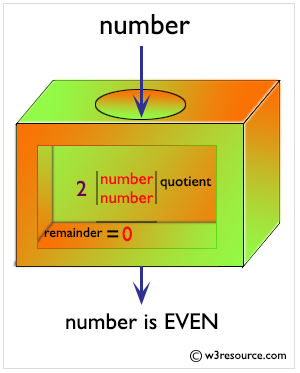Pictorial Presentation of Odd Numbers: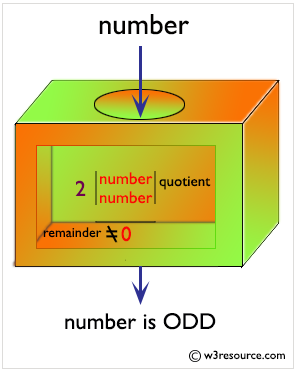Sample Solution:

Python Code:

numbers = (1, 2, 3, 4, 5, 6, 7, 8, 9) # Declaring the tuple
count_odd = 0
count_even = 0
for x in numbers:
if not x % 2:
count_even+=1
else:
count_odd+=1
print("Number of even numbers :",count_even)
print("Number of odd numbers :",count_odd)

Sample Output:

Number of even numbers : 4
Number of odd numbers : 5

Flowchart: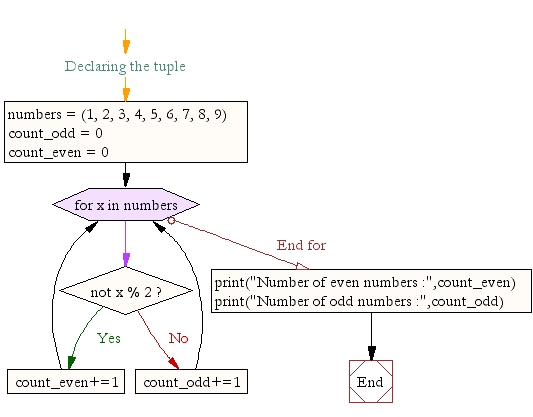Even Numbers between 1 to 100: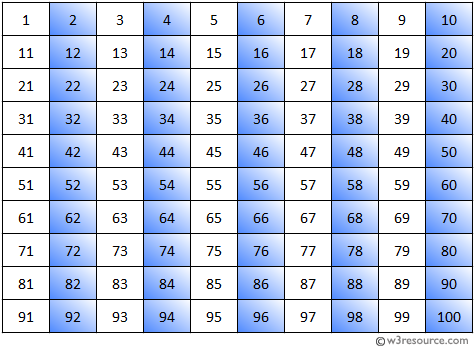Odd Numbers between 1 to 100: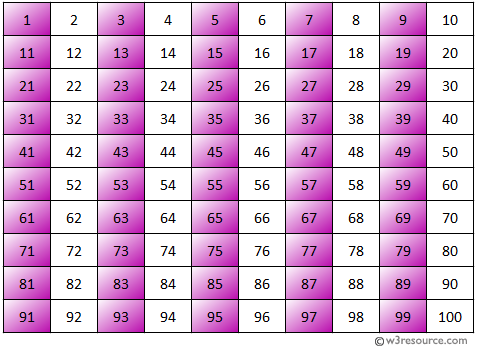Visualize Python code execution:

The following tool visualize what the computer is doing step-by-step as it executes the said program:

Python Code Editor:

Have another way to solve this solution? Contribute your code (and comments) through Disqus.

What is the difficulty level of this exercise?

Test your Programming skills with w3resource's quiz.

﻿

Python: Tips of the Day

Time it:

timeit library is great for timing Python execution times. Just pass a function in string format to timeit.timeit() method and it will carry out 1 million executions to report the minimum time the code takes.

Its very useful to compare small code pieces and different functions but can be sluggish with big code.

Check out the example below demonstrating the execution time difference between 2 very similar list comprehension methods in Python:

import timeit
lst1='''list(range(100))'''
lst2='''[i for i in range(100)]'''
a=timeit.timeit(lst1)
b=timeit.timeit(lst2)
print(a, b, sep="------")

Output:

1.9301698543131351------5.389458132907748